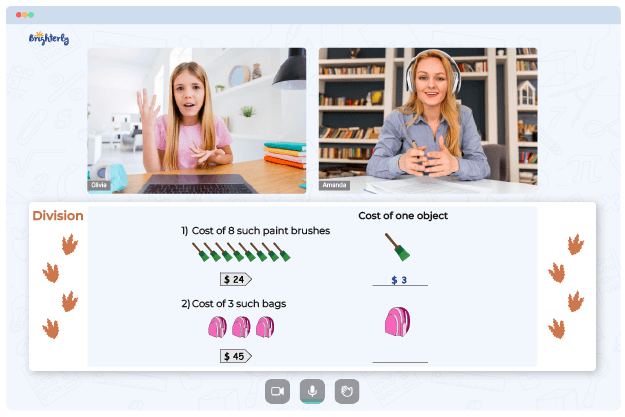# Dividing Integers Worksheets

The division of integers worksheet is helpful as it contains division integers exercises for 7th graders. These exercises cover division operations that progress into two-digits integers from the positive and negative quotients. Consistent practice will allow kids to apply the integer division rules to some basic division exercises.

## Benefits of a Dividing Integers Worksheet 7th Grade

The knowledge of division Integers operation is significant for a child who wants to understand math concepts generally. These worksheets contain numerous division integers activities that help understand and strengthen a kid’s division skills.

Math for Kids

Is Your Child Struggling With Math?
1:1 Online Math TutoringThese worksheets give children the freedom and flexibility to study at will as the tasks are highly interactive. They make working on basic division integers’ operations an effortless and enjoyable process for children.

The dividing integers worksheet grade 7 students use in the classroom include primary concepts teachers typically discuss in their lectures. Therefore, the resources are also a delight for teachers because they make lecturing easier.### Dividing Integers Worksheets PDF

Dividing Integers Worksheet### Dividing Integers Worksheets PDF### Dividing Integers Worksheets PDF

Division Of Integers Worksheet### More Division Worksheets

Struggling with Division?• Does your child struggle with understanding division?
• Try lessons with an online tutor.

Is your child having difficulties mastering the concept of division? An online tutor could provide the necessary support.